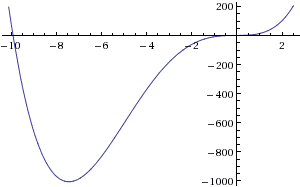# End behaviour of functions

zeion

## Homework Statement

My textbook tells me that "An even-degree polynomial function has the same end behavior" and "An odd-degree polynomial polynomial function has opposite end behaviors".

Why is it that when I try to graph let's say "x^4 + 10x^3 + x^2 +x + 1" the end behaviors are opposite even though the function is an even-degree polynomial function?

Staff Emeritus
Homework Helper
Gold Member

## Homework Statement

My textbook tells me that "An even-degree polynomial function has the same end behavior" and "An odd-degree polynomial polynomial function has opposite end behaviors".

Why is it that when I try to graph let's say "x^4 + 10x^3 + x^2 +x + 1" the end behaviors are opposite even though the function is an even-degree polynomial function?

## The Attempt at a Solution

Perhaps it's because you are graphing it over an itnerval which doesn't extend far enough.

Here's a graph of your polynomial for -10.1 ≤ x ≤ 2.5 . (from WolframAlpha)#### Attachments

zeion
Oh how silly of me. Thanks.